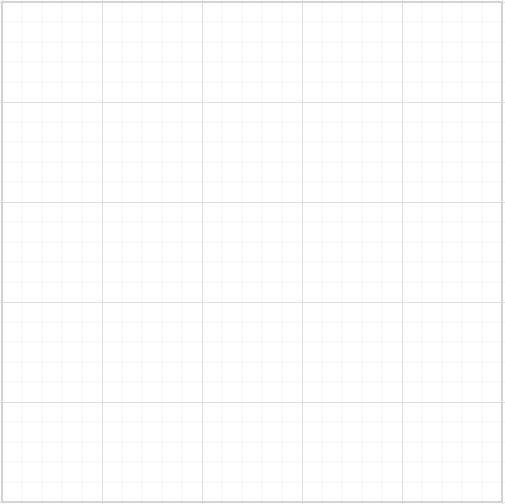# Model

The Diagram model represents the data to render the Diagram and to manipulate the Diagram elements. The following code illustrates how to define Diagram model.

• aspx-cs
• ``````<ej:Diagram runat="server" ClientIDMode="Static" ID="DiagramWebControl" Width="100%" Height="100%">
<PageSettings PageWidth="2000" PageHeight="2000"/>
</ej:Diagram>``````To explore more model properties, refer to Model Properties.